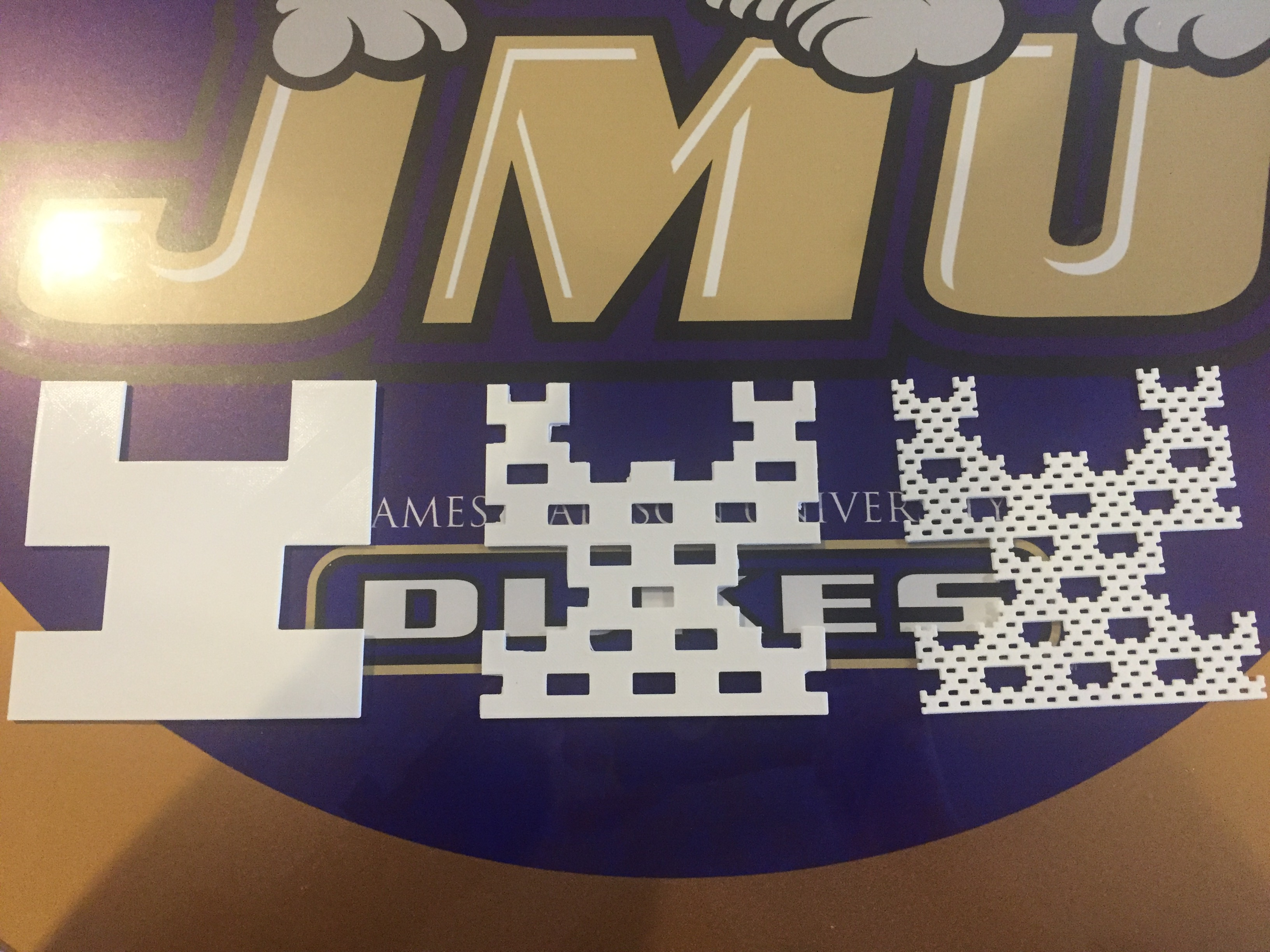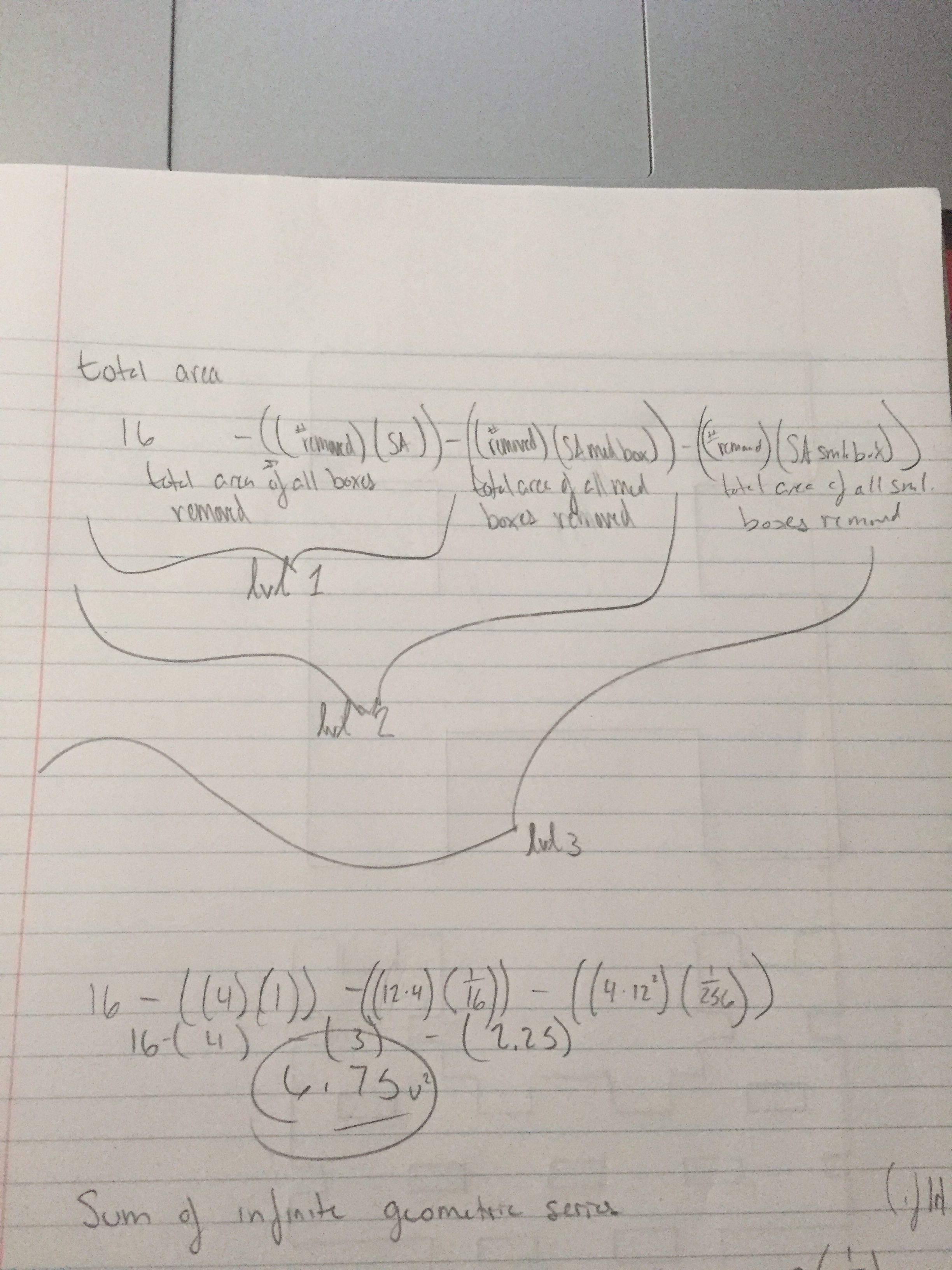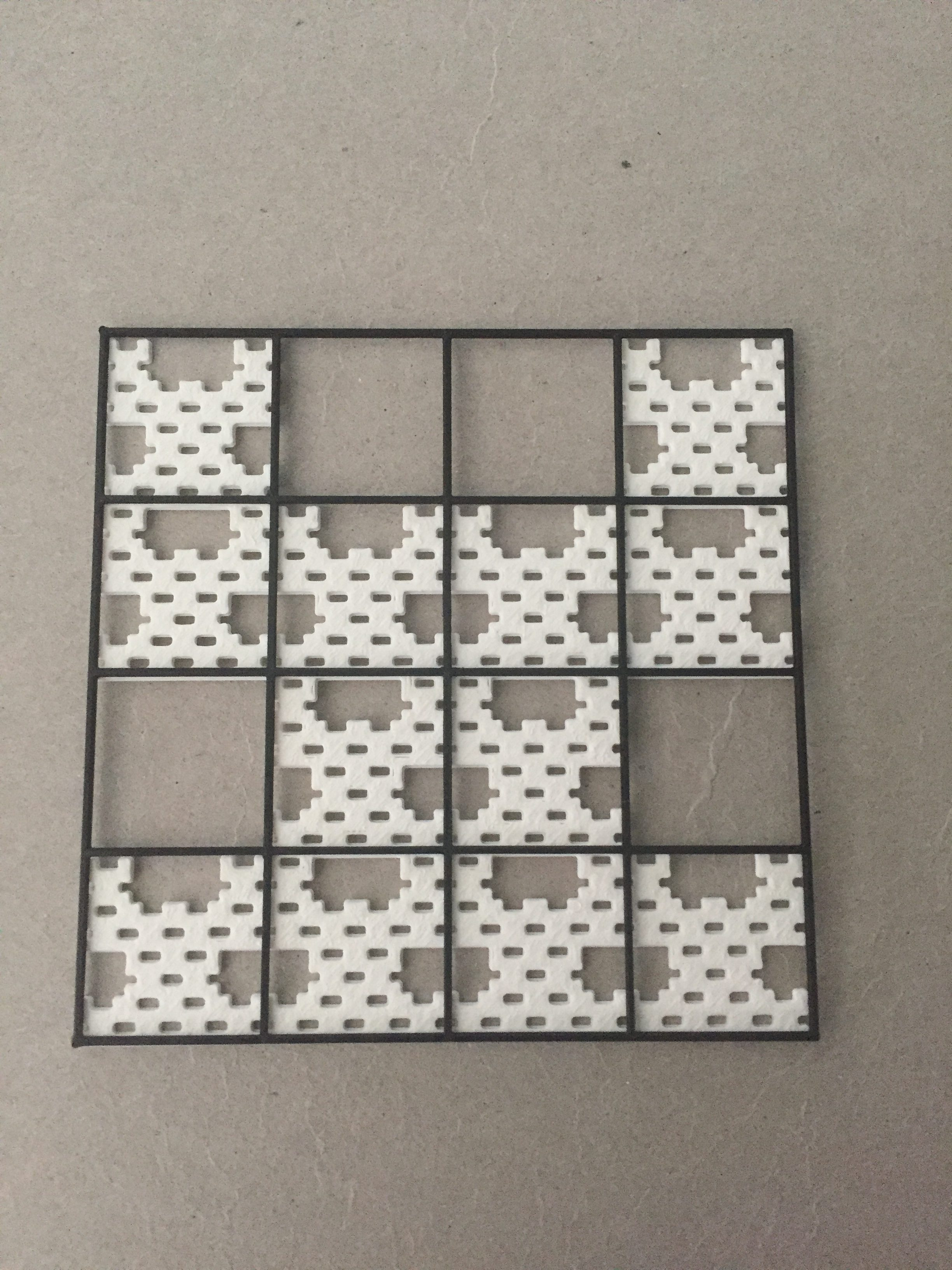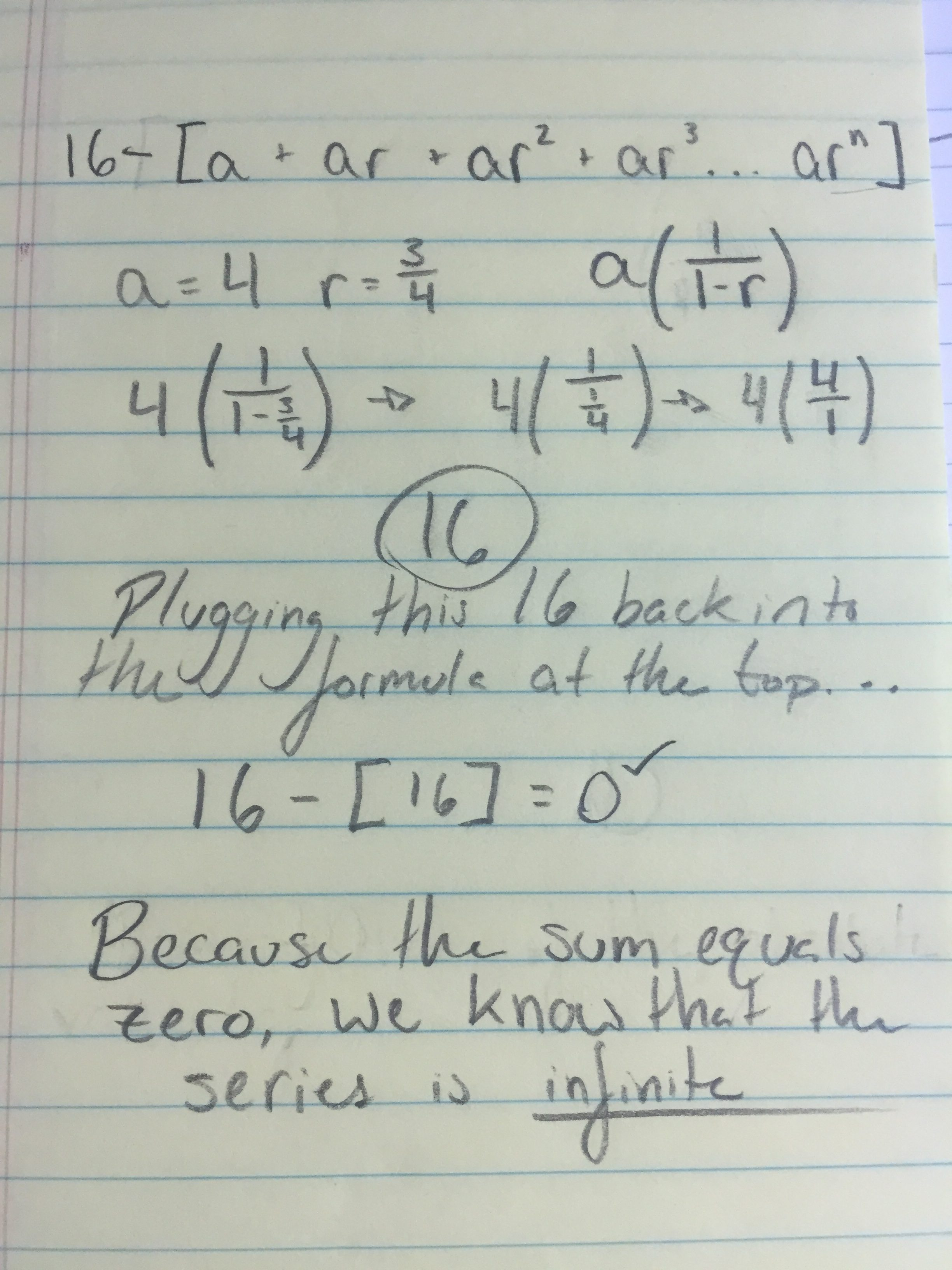I named my fractal the Space Invader because the white color and shape reminds me of the aliens from the classic arcade game Space Invaders. When designing this fractal carpet, I did not have any specific shape in mind, but I knew I wanted a symmetrical fractal to work with. After removing the squares from points (0,1), (3,1), (1,3), and (2,3) I found a shape I was ready to work with. Calculating the surface area of Level 1 was fairly easy, seeing that I only had to subtract 4 from the total of 16. After that, Level 2 was my next problem to tackle. Because the smaller squares have an area of 1, the subtracted part of those smaller squares would be equal to 1/16. Multiplying 4, 12, and 1/16 and subtracting it from the area of Level 1 gave me the area for Level 2, which is 9. Level 3 was simple after finding Level 2. I needed to again multiply by 12 because there were now 12 smaller whole squares I was using, and multiply again by 1/16 due to the fact that these squares were getting smaller at a constant rate. Multiplying 4, 144, and 1/256 and again subtracting from the previous level gave me the area of Level 3, which ended up being 6.75. The picture below shows my work. Also included below is a picture of my Level 3 with the gridlines of a fully intact Level 1.The most difficult part of my calculations was easily finding the sum of the geometric series.

In order to do this I needed to use the formula   a(1/1-r). In my case, a=4 (the number of squares I had originally removed), and r= 3/4 (because, after removing 4 from 16, we are left with 12/16 which simplifies to 3/4).

The work below illustrates my process: